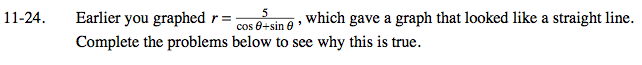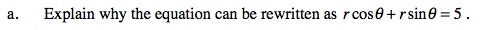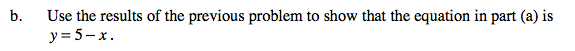Home > PC > Chapter 11 > Lesson 11.1.2 > Problem11-24

11-24.
1. Earlier you graphed r =, which gave a graph that looked like a straight line. Complete the problems below to see why this is true. Homework Help ✎

1. Explain why the equation can be rewritten as rcosθ + rsinθ = 5.

2. Use the results of the previous problem to show that the equation in part (a) is y = 5 − x.Multiply both sides of the equation by (cosθ + sinθ).In problem 11-23 you determined that x = ? and y = ?